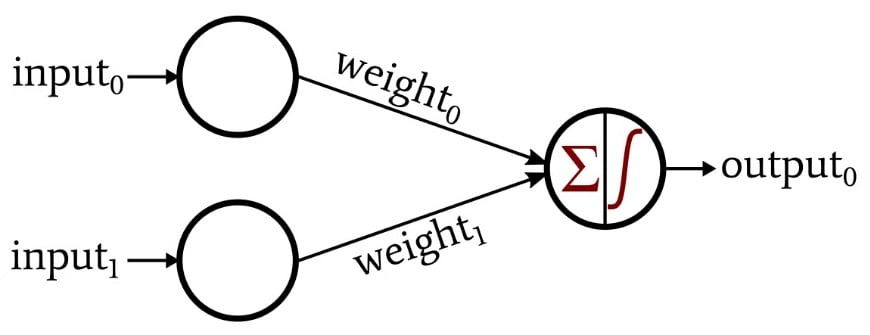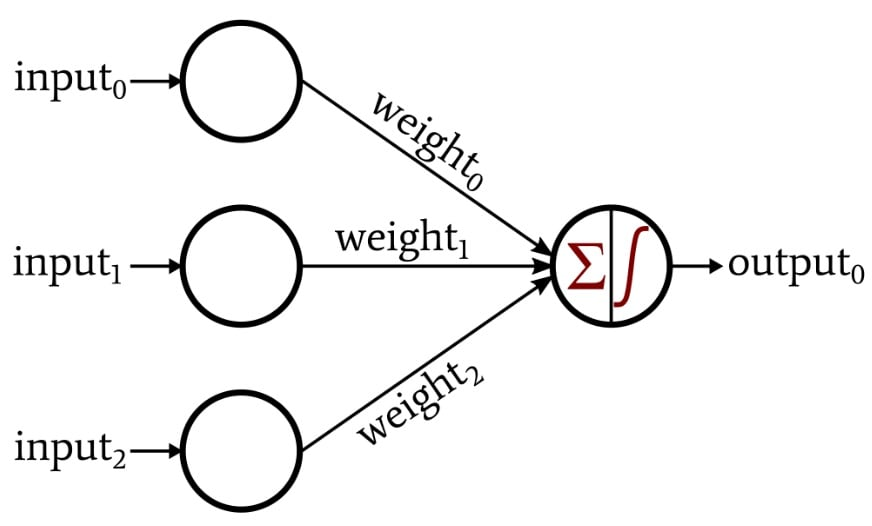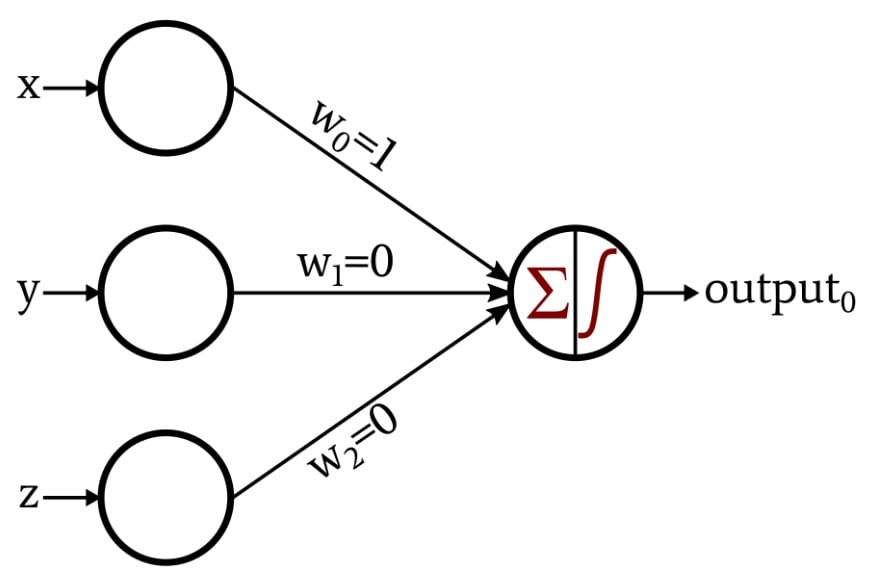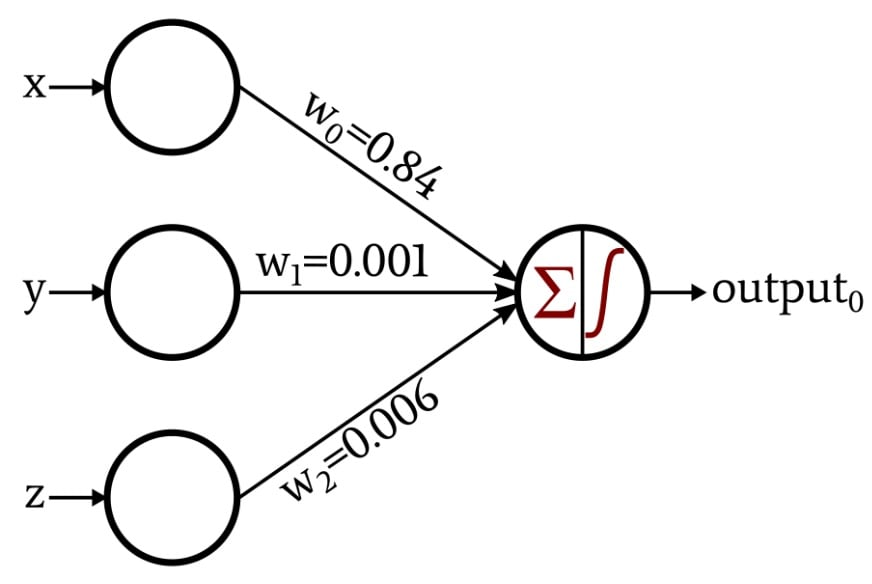Technical Article

# How to Use a Simple Perceptron Neural Network Example to Classify Data

November 17, 2019 by Robert Keim

## This article demonstrates the basic functionality of a Perceptron neural network and explains the purpose of training.

If you'd like to start from the beginning or jump ahead, you can check out the other articles here:

### What Is a Single-Layer Perceptron?

In the previous article, we saw that a neural network consists of interconnected nodes arranged in layers. The nodes in the input layer distribute data, and the nodes in other layers perform summation and then apply an activation function. The connections between these nodes are weighted, meaning that each connection multiplies the transferred datum by a scalar value.Note that this configuration is called a single-layer Perceptron. Yes, I know, it has two layers (input and output), but it has only one layer that contains computational nodes.

### Classifying with a Perceptron

In this article, we’ll explore Perceptron functionality using the following neural network.As you can see, our input dimensionality is three. We can think of this Perceptron as a tool for solving problems in three-dimensional space. For example, let’s propose the following problem: If a point in three-dimensional space is located below the x-axis, it corresponds to an invalid datum. If the point is on or above the x-axis, it corresponds to a valid datum that must be retained for further analysis. We need this neutral network to categorize our data, with an output value of 1 indicating a valid datum and a value of 0 indicating an invalid datum.

First, we must map our three-dimensional coordinates to the input vector. In this example, input0 is the x component, input1 is the y component, and input2 is the z component. Next, we need to determine the weights. This example is so simple that we don’t need to train the network. We can simply think about the required weights and assign them:All we need to do now is specify that the activation function of the output node is a unit step expressed as follows:

$f(x)=\begin{cases}0 & x < 0\\1 & x \geq 0\end{cases}$

The Perceptron works like this: Since w1 = 0 and w2 = 0, the y and z components make no contribution to the summation generated by the output node. The only input datum that affects the summation is the x component, which is delivered to the output node unmodified because w0 = 1. If the point in three-dimensional space is below the x-axis, the output node’s summation will be negative, and the activation function will convert this negative value into output0 = 0. If the point in three-dimensional space is on or above the x-axis, the summation will be equal to or greater than zero, and the activation function will convert this into output0 = 1.

### Solving Problems with a Perceptron

In the previous section, I described our Perceptron as a tool for solving problems. You may have noticed, though, that the Perceptron didn’t do much problem solving—I solved the problem and gave the solution to the Perceptron by assigning the required weights.

At this point we have reached a crucial neural-network concept: I was able to quickly solve the valid/invalid classification problem because the relationship between the input data and the desired output values is very simple. However, in many real-life situations, it would be extremely difficult for a human being to formulate a mathematical relationship between input data and output values. We can acquire input data and we can record or produce corresponding output values, but we don’t have a mathematical route from input to output.

A helpful example is handwriting recognition. Let’s say that we have images of handwritten characters, and we want to categorize those images as “a,” “b,” “c,” etc., so that we can convert handwriting into normal computer text. Anyone who knows how to write and read will be able to generate input images and then assign correct categories to each image. Thus, collecting input data and corresponding output data is not difficult. On the other hand, it would be exceedingly difficult to look at the input-output pairs and formulate a mathematical expression or algorithm that would correctly convert input images into an output category.

Thus, handwriting recognition and many other signal-processing tasks present mathematical problems that human beings cannot solve without the help of sophisticated tools. Despite the fact that neural networks can’t think and analyze and innovate, they allow us to solve these difficult problems because they can do something that human beings can’t—that is, rapidly and repeatedly perform calculations involving potentially immense amounts of numerical data.

Training the Network

The process that allows a neural network to create a mathematical pathway from input to output is called training. We give the network training data consisting of input values and corresponding output values, and it applies a fixed mathematical procedure to these values. The goal of this procedure is to gradually modify the network’s weights such that the network will be able to calculate correct output values even with input data that it has never seen before. It’s essentially finding patterns in the training data and generating weights that will produce useful output by applying these patterns to new data.

The following diagram shows the valid/invalid classifier discussed above, but the weights are different. These are weights that I generated by training the Perceptron with 1000 data points. As you can see, the training process has allowed the Perceptron to automatically approximate the mathematical relationship that I identified through human-style critical thinking.### In the Next Article…

I’ve shown you the results of training this Perceptron, but I haven’t said anything about how I obtained these results. The next article will describe a short Python program that implements a single-layer Perceptron neural network, and I will also explain my training procedure.